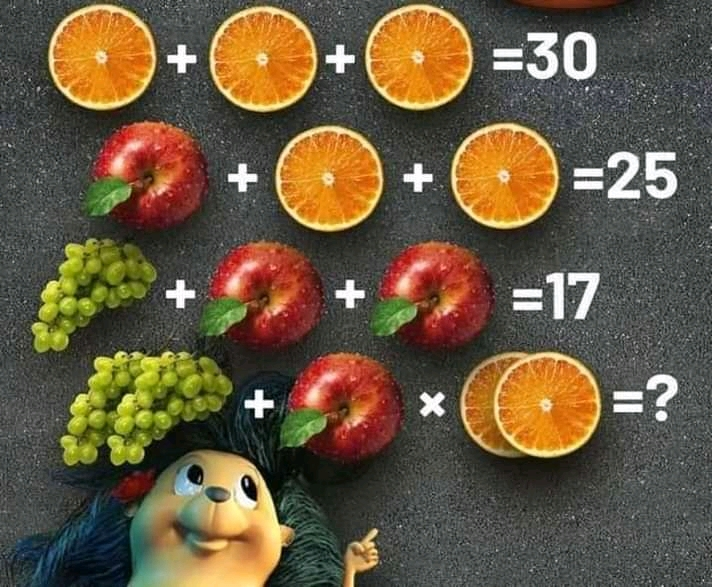# Answer: Orange slice apple grape math puzzle# Answer: Orange slice apple grape math puzzle

The  Orange slice apple grape math puzzle Is one of those fruit math  puzzles that keeps many people scratching their heads on the internet, especially on Twitter, reddit, WhatsApp and Facebook.  I saw  the Puzzle which was published on Facebook, many people solved it and had different opinions and answers. Today I will give you the correct answer to the puzzle with solving the details.

## Two bunch of grapes + apple × two slices of Orange = ?

The answer would be 114, the trick in the fruit math Puzzle is found in the last equation where BODMAS needs to be applied. Check the solution below.

Also check: How many eggs can you see picture Puzzle answer.

Here's the puzzle extraction:

If

A slice of Orange + A slice of Orange

A slice of Orange= 30,

Apple + Apple + Apple = 30,

Apple + A slice of Orange

+ A slice of Orange

= 25,

Bunch of grapes + apple + apple= 17

then,

Two bunch of grapes + apple × two slices of Orange = ?

From the extraction above, you can easily get the correct answer.

Due to the Corona various pandemic, many people isolate themselves because of lockdowns and to avoid contracting or spreading the deadly disease.

Isolation can lead to boredom,  one way to refresh the brain, relax and get busy is  by solving Math puzzles and Quizzes.

Many game lovers like solving math puzzles suduku, quizzes like this one to refresh their brain.

I will solve each equation and then substitute answers in the final equation and simplify applying BODMAS and making "subjects of the formula" in some cases.

Equation one

3 Orange Slices =30

Implies Orange Slice  = 30÷3,

Orange slice  = 10.

Equation two

Apple + 10 + 10 = 25

Apple = 25 - 20

Apple = 5.

Equation three

Bunch of grapes + 5 + 5 = 17

Bunch of grapes = 17 - 10

Bunch of grapes = 7

Therefore, the value of the variables are as Thus:

Orange Slice= 10

Apple = 5

Bunch of grapes =7

I will substitute in the final equation

Final equation

I will substitute the values of the variables above in the final equation below and simplify.

Two bunch of grapes + apple × two slices of Orange = ?

2(7) + 5 × 2(10) =?

14 + 5 × 20 = ?

14 + 100 = ?

114 = ?

So the correct answer to the tricky  math Puzzle: Two bunch of grapes + apple × two slices of Orange = ?

Is

= 114.

Do you have another way to arrive at this Answer? Share with us.

Don't forget to test your friends, family members and loved ones by sharing the puzzle.